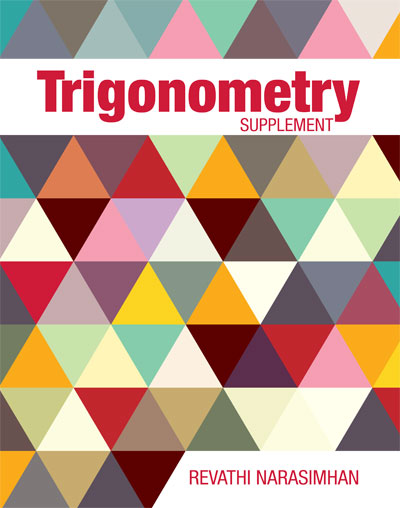## This is a small three chapter booklet can supplement many different courses.

Trigonometric Functions
1.1  Angles and Their Measures
1.2  Trigonometric Functions Using the Unit Circle
1.3  Right Triangle Trigonometry
1.4  Trigonometric Functions of Any Angle Using Right Triangles
1.5  Graphs of Sine and Cosine Functions
1.6  Graphs of Other Trigonometric Functions
1.7  Inverse Trigonometric Functions

Trigonometric Identities and Equations
2.1  Verifying Identities
2.2  Sum and Difference Identities
2.3  Multiple-Angle Identities; Sum and Product Identities
2.4  Trigonometric Equations